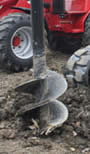# Area of a Circle

## Calculator

Enter the radius, diameter, circumference or area of a Circle to find the other three. The calculations are done "live":

images/circle-dia-circ.js

## How to Calculate the Area

The area of a circle is:

π (Pi) times the Radius squared:A = π r2
or, when you know the Diameter:A = (π/4) × D2
or, when you know the Circumference:A = C2 / 4π

### Example: What is the area of a circle with radius of 3 m ?Area= π r2
= π × 32
= 3.14159... × (3 × 3)
= 28.27 m2 (to 2 decimal places)

## How to Remember?(even though pies are usually round)

## Comparing a Circle to a Square

It is interesting to compare the area of a circle to a square:A circle has about 80% of the area of a similar-width square.
The actual value is (π/4) = 0.785398... = 78.5398...%

Why? Because the Square's Area is w2
and the Circle's Area is (π/4) × w2

### Example: Compare a square to a circle of width 3 m

Square's Area = w2 = 32 = 9 m2

Estimate of Circle's Area = 80% of Square's Area = 80% of 9 = 7.2 m2

Circle's True Area = (π/4) × D2 = (π/4) × 32 = 7.07 m2 (to 2 decimals)

The estimate of 7.2 m2 is not far off 7.07 m2

## A "Real World" Example

### Example: Max is building a house. The first step is to drill holes and fill them with concrete.

The holes are 0.4 m wide and 1 m deep, how much concrete should Max order for each hole?The holes are circular (in cross section) because they are drilled out using an auger.

The diameter is 0.4m, so the Area is:A = (π/4) × D2

A = (3.14159.../4) × 0.42

A = 0.7854... × 0.16

A = 0.126 m2 (to 3 decimals)

And the holes are 1 m deep, so:

Volume = 0.126 m2 × 1 m = 0.126 m3

So Max should order 0.126 cubic meters of concrete to fill each hole.

Note: Max could have estimated the area by:

• 1. Calculating a square hole: 0.4 × 0.4 = 0.16 m2
• 2. Taking 80% of that (estimates a circle): 80% × 0.16 m2 = 0.128 m2
• 3. And the volume of a 1 m deep hole is: 0.128 m3

And something interesting for you: